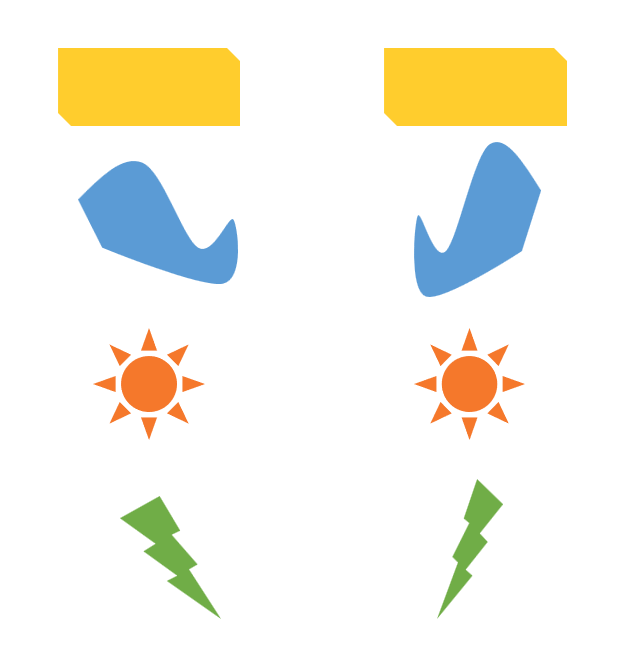Doubtnut App - Get 50 Lakh+ video solutions better than GoogleInstall Now

# Chapter 7 CONGRUENCE OF TRIANGLES Class 7 MATHS Ncert Solutions

Baccho, hum apni day to day life m aise bohot si shapes and figures ko dekhte hai jo ki exactly same hoti hai. For example, two 1 rupee coins will have the same shape and size but a 1 rupee and a 2 rupee coin might have different sizes and would not overlap each other completely. Jo shapes ek dusre ko completely overlap krti hai unhe congruent shapes kaha jaata hai. Let's play a quick game to understand this better. Look at the following pair of shapes and identify which all are congruent figures.In geometry, congruent means identical in shape and size. Congruence can be applied to line segments, angles, and figures. Any two line segments are said to be congruent if they are equal in length. Two angles are said to be congruent if they are of equal measure. Two triangles are said to be congruent if their corresponding sides and angles are equal. Baccho, aapne apne previous chapter m triangles aur unki properties k baare m pdha tha. Jab do triangles ki saari properties jaise ki sides, angles, vertices, etc. same hoti hai to unhe congruent triangles kaha jaata hai. Iss chapter m hum congruent triangles k baare m detail m pdhenge. Hum alag alag criterias k baare m seekhenge jinse triangles m congruency define ho skti hai. So let's begin.